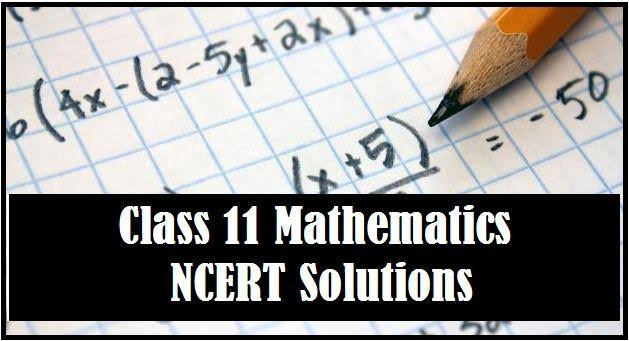Online Coaching Classes, CBSE Online Maths Coaching Classes NCERT Solutions For Class 11th Maths with All Chapters! -Share us on
eMaths provide all accurate and detailed solutions to the class 11 Maths NCERT exercise questions. All these questions are solved by our best mathematics teachers, who are highly experienced in the field of CBSE board education. The prescribed textbooks by NCERT are commonly used by all schools that are affiliated to the Central Board of Secondary Education. The class 11 Maths NCERT solutions not only helps in your school level exam preparation but also in other different entrance exams. Moreover, it also covers all the exercise problems of all the chapters of the class 11th NCERT Maths book. All the solutions of questions are explained in a proper manner so students are enabled to understand them. In addition, the most complex problems are categorically broken down to make learning easier. All the solutions are 100 % accurate according to NCERT guideline and syllabus. eMaths brings the accurate and comprehensive solutions for all questions given in NCERT class 11 maths book. All these questions are solved by the experts. The step-wise solutions will help the students to easily understand the concepts and formulas. Let’s have a look at the following features of class 11 Maths NCERT solutions: Each solution is structured in step wise form. All solutions are explained by subject matter experts. Each solution is strictly according to the guidelines followed in the CBSE marking scheme for exams. Available in PDF format which can be downloaded free of cost. The NCERT Solutions for class 11th Maths consist all the chapter-wise solutions of questions, which is a great material for students who are preparing for class 11 exams. The chapters covered here are trigonometric functions, sequence and series, relations and functions, three-dimensional geometry, binomial theorem, probability and others. Here, all the solutions are presented in the form of PDF, which is easy to download and avail the students to find the answers conveniently according to their respective questions chapter-wise. Find below the links to download the NCERT solutions for all chapter of CBSE Class 11 Maths:
 Chapter No. Chapter Name Download Link 1. Sets 2. Relations And Functions 3. Trigonometric Function 4. Principle of Mathematical Induction 5. Complex Numbers 6. Liner Inequation 7. Permutation And Combination 8. Biomial Theorem 9. Sequence And Series 10. Straight Lines 11. Conic Section 12. Limits And Derivatives 13. Mathematical Reasoning 14. Statistics
Whenever students face any problem in understanding the solution of any question then they can contact the eMaths experts. They will ensure that you understand the problem properly enabling you to solve a problem based on the same concept easily on the day of the examination.